Smartick is an advanced online program that teaches kids math and coding in only 15 min. a day

Mar12

# Using the LCM (Least Common Multiple) to Solve Problems

In a previous post, we looked at the Least Common Multiple and how to calculate it.

Today we’re going to look at a couple of examples in which we use the Least Common Multiple (LCM) to solve math problems. In one example, we’ll use the LCM to calculate the answer directly, and in the other we’ll need it as a step to find the solution to the problem.

###The problem tells us that there are the same number of gold coins on each island, but distributed in different ways. On one of the islands, Buck the Buccaneer will find the coins in chests of 8. On the other, Piper the Pirate will find them in chests of 36.

We know that if the pirates add their respective coins 8 by 8, and 36 by 36, the numbers they’ll get when they finish adding will be the same, because there are the same number of coins on each island. To put it another way, the number of coins on each island must be a multiple of both 8 and 36, and it could be any number that meets this condition.

But the statement asks us to work out the minimum number of coins they’ll find. In other words, the smallest of all the common multiples of 8 and 36. Therefore, we’re talking about the LCM of 8 and 36.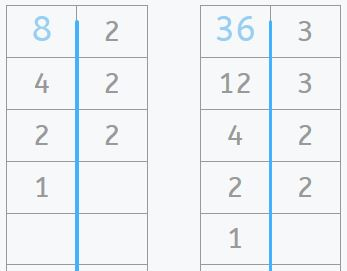###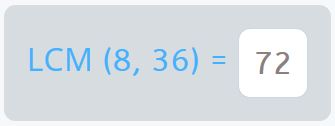So Buck the Buccaneer and Piper the Pirate will find at least 72 gold coins on each island.

### Using the LCM as a step towards solving a problem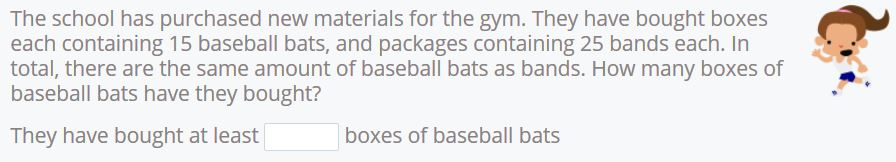This time the problem asks us to work out the least possible number of boxes of baseball bats that they must have bought.

To work out the answer, first we need to calculate the smallest possible total number of baseball bats that they could have purchased. They bought the baseball bats in boxes of 15, and bands in boxes of 25. We also know that they’ve bought the same number of baseball bats as rubber bands. Therefore, the minimum number of baseball bats that they could have bought is the LCM of 15 and 25.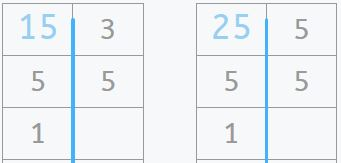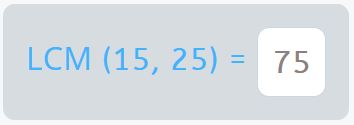Now we know that they must have bought at least 75 baseball bats, and they have the same number of bats as bands.

The only thing left to do is divide the number of bats by the amount of bats in each box to work out the smallest possible number of boxes of baseball bats they’ve bought.That’s it! They’ve bought at least 5 boxes of baseball bats.

If you want to keep working on these types of exercises and many more, log in to Smartick and try it for free.